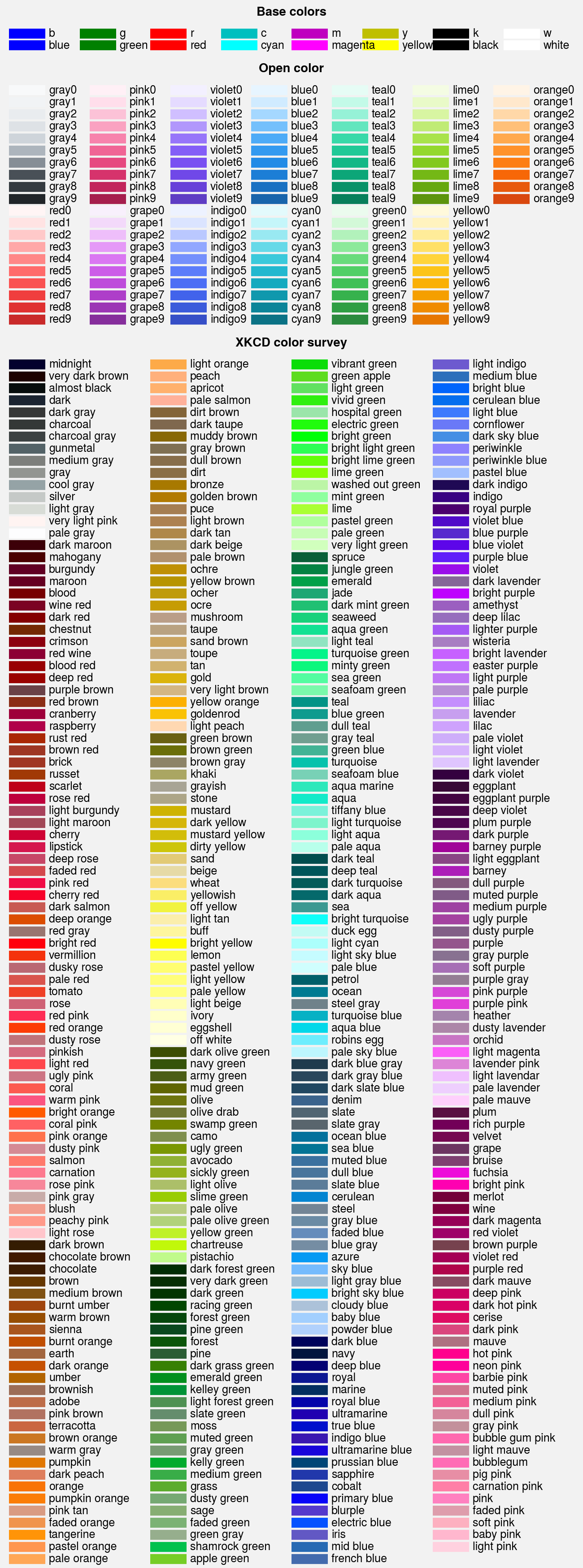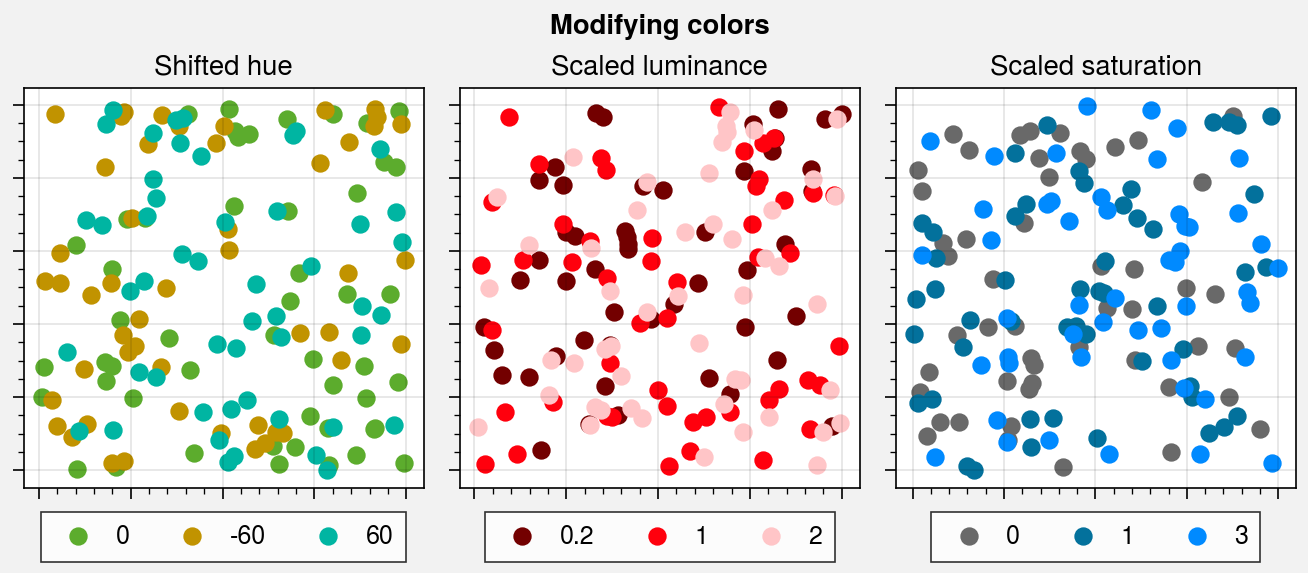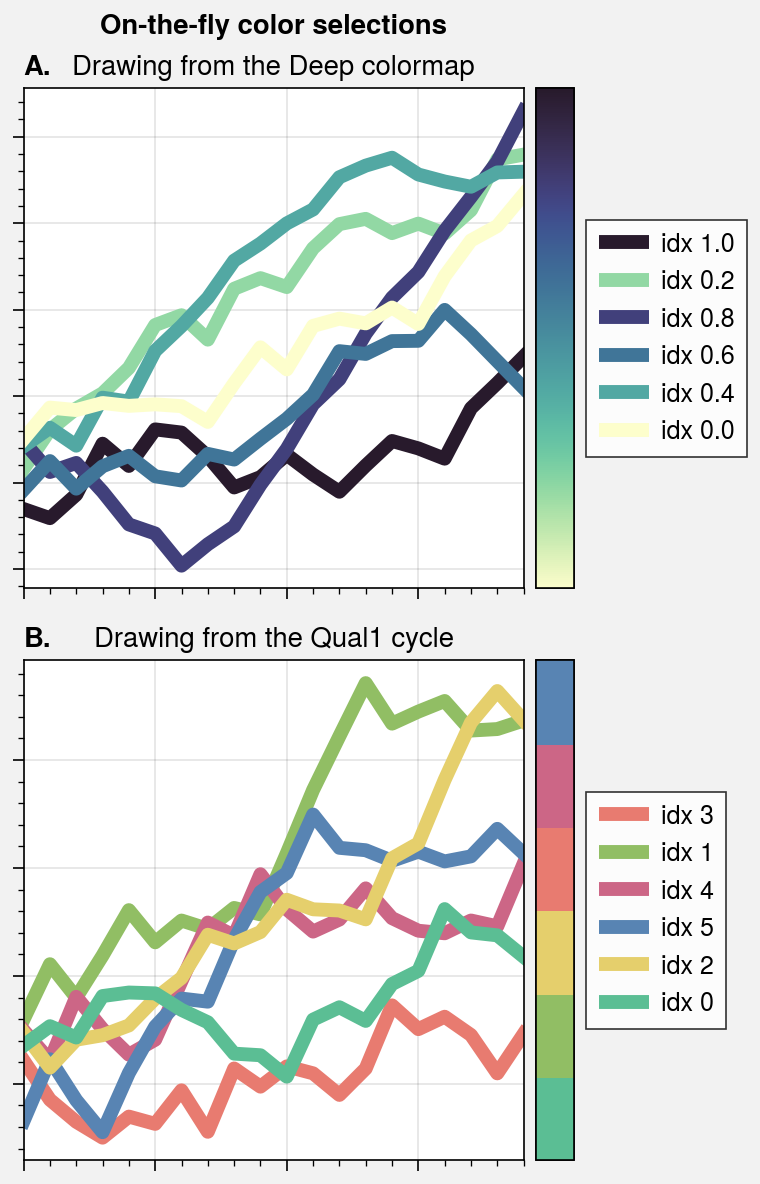# Color names¶

ProPlot registers several new color names and includes tools for defining your own color names. These features are described below.

## Included colors¶

ProPlot adds new color names from the XKCD color survey and the Open Color UI design color palettes. You can use show_colors to generate a table of these colors. Note that matplotlib’s native X11/CSS4 named colors are still registered, but some of these color names may be overwritten by the XKCD names, and we encourage choosing colors from the below tables instead. ProPlot registers the XKCD names because the selection is larger and the names are more likely to match your intuition for what a color “should” look like.

To reduce the number of registered color names to a more manageable size, ProPlot filters the available XKCD colors so that they are sufficiently distinct in the perceptually uniform colorspace. This makes it a bit easier to pick out colors from the table generated with show_colors. Similar names were also cleaned up – for example, 'reddish' and 'reddy' are changed to 'red'.

:

import proplot as pplt
fig, axs = pplt.show_colors()## Modifying colors¶

You can quickly modify colors using the set_hue, set_saturation, set_luminance, shift_hue, scale_saturation and scale_luminance functions. The set functions change individual hue, saturation, or luminance values in the perceptually uniform colorspace specified by the space keyword. The scale functions shift or scale the hue, saturation, or luminance by the input value – for example, scale_luminance('color', 1.2) makes 'color' color 20% brighter. These are useful for creating color gradations outside of Cycle or if you simply spot a color you like and want to make it a bit brighter, less vibrant, etc.

:

import proplot as pplt
import numpy as np

# Figure
state = np.random.RandomState(51423)
fig, axs = pplt.subplots(ncols=3, axwidth=2)
axs.format(
suptitle='Modifying colors',
toplabels=('Shifted hue', 'Scaled luminance', 'Scaled saturation'),
toplabelweight='normal',
xformatter='none', yformatter='none',
)

# Shifted hue
with pplt.rc.context({'legend.handlelength': 0}):
N = 50
marker = 'o'
for shift in (0, -60, 60):
x, y = state.rand(2, N)
color = pplt.shift_hue('grass', shift)
axs.scatter(x, y, marker=marker, c=color, legend='b', label=shift)

# Scaled luminance
for scale in (0.2, 1, 2):
x, y = state.rand(2, N)
color = pplt.scale_luminance('bright red', scale)
axs.scatter(x, y, marker=marker, c=color, legend='b', label=scale)

# Scaled saturation
for scale in (0, 1, 3):
x, y = state.rand(2, N)
color = pplt.scale_saturation('ocean blue', scale)
axs.scatter(x, y, marker=marker, c=color, legend='b', label=scale)## Colors from colormaps¶

If you want to draw an individual color from a colormap or a color cycle, use color=(cmap, coord) or color=(cycle, index) with any command that accepts the color keyword. The coord should be between 0 and 1, while the index is the index on the list of cycle colors. This feature is powered by the ColorDatabase class. This is useful if you spot a nice color in one of the available colormaps or color cycles and want to use it for some arbitrary plot element. Use the to_rgb or to_rgba functions to retrieve the RGB or RGBA channel values.

:

import proplot as pplt
import numpy as np

# Figure
fig, axs = pplt.subplots(nrows=2, share=0)
axs.format(
xformatter='null', yformatter='null', abc=True, abcloc='l', abcstyle='A.',
suptitle='On-the-fly color selections'
)

# Drawing from colormaps
ax = axs
name = 'Deep'
idxs = pplt.arange(0, 1, 0.2)
state = np.random.RandomState(51423)
state.shuffle(idxs)
for idx in idxs:
data = (state.rand(20) - 0.4).cumsum()
h = ax.plot(
data, lw=5, color=(name, idx),
label=f'idx {idx:.1f}', legend='r', legend_kw={'ncols': 1}
)
ax.colorbar(pplt.Colormap(name), loc='r', locator='none')
ax.format(title=f'Drawing from the {name} colormap', grid=True)

# Drawing from color cycles
ax = axs
name = 'Qual1'
idxs = np.arange(6)
state.shuffle(idxs)
for idx in idxs:
data = (state.rand(20) - 0.4).cumsum()
h = ax.plot(
data, lw=5, color=(name, idx),
label=f'idx {idx:.0f}', legend='r', legend_kw={'ncols': 1}
)
ax.colorbar(pplt.Colormap(name), loc='r', locator='none')
ax.format(title=f'Drawing from the {name} cycle')You can register your own colors by adding .txt files to the ~/.proplot/colors directory and calling register_colors. This command is also called on import. Each file should contain lines that look like color: #xxyyzz where color is the registered color name and #xxyyzz is the HEX color value. Lines beginning with # are ignored as comments.